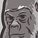Announcements
You can succeed best and quickest by helping others to succeed. Join the conversation.
cancel
Showing results for
Did you mean:Creator III

## help with expression

I have following expression on my table . all i want is to show all the amount by the each see date ,  currently i have to select see date to see the value and it will only show the value of selected date, but i want this to be display all by the see date .

dimension is see date

Sum({<[Request Type]={'SA'},[ Action]={'APP'},[Dep Date]={">\$(=[see Date])"},[T Date]={"<\$(=[see Date])"}>}[Amount])
-
Sum({<[Request Type]={'CR'},[ Action ]={'APP'},[ Dep Date]={">\$(=[see Date])"},[T Date]={"<\$(=[see Date])"}>}[Amount])

Labels (1)
• ### expression

1 Solution

Accepted SolutionsMVP
Not sure, why this got changed. I just mentioned <= and >= like below
Sum({<[Request Type]={'SA'},[ Action]={'APP'},[Dep Date]={"=[Dep Date]>[see Date]"},[T Date]={"=[T Date]<[see Date]"}>}[Amount])
-
Sum({<[Request Type]={'CR'},[ Action ]={'APP'},
[Dep Date]={"=[Dep Date]>[see Date]"},[T Date]={"=[T Date]<[see Date]"}>}[Amount])
Before develop something, think If placed (The Right information | To the right people | At the Right time | In the Right place | With the Right context)
5 RepliesMVP
For me the expression what you did is quiet wrong, perhaps this? I am just altering your expression to proper syntax

Sum({&lt;[Request Type]={'SA'},[ Action]={'APP'},[Dep Date]={"=[Dep Date]&gt;[see Date]"},[T Date]={"=[T Date]&lt;[see Date]"}&gt;}[Amount])
-
Sum({&lt;[Request Type]={'CR'},[ Action ]={'APP'},
[Dep Date]={"=[Dep Date]&gt;[see Date]"},[T Date]={"=[T Date]&lt;[see Date]"}&gt;}[Amount])
Before develop something, think If placed (The Right information | To the right people | At the Right time | In the Right place | With the Right context)Creator III
Author

what is &lt; does in this expression .MVP
Not sure, why this got changed. I just mentioned <= and >= like below
Sum({<[Request Type]={'SA'},[ Action]={'APP'},[Dep Date]={"=[Dep Date]>[see Date]"},[T Date]={"=[T Date]<[see Date]"}>}[Amount])
-
Sum({<[Request Type]={'CR'},[ Action ]={'APP'},
[Dep Date]={"=[Dep Date]>[see Date]"},[T Date]={"=[T Date]<[see Date]"}>}[Amount])
Before develop something, think If placed (The Right information | To the right people | At the Right time | In the Right place | With the Right context)Creator III
Author

Thanks @Anil_Babu_Samineni

Its gives the same result. but the value only shows when i click viewdate from listbox where as i am trying to show every viewdate value in table .  is it because VIewDate is independent island in data model  which is not linked to any table?

Thanks

AgainMVP
If that table is not associated to any, definitely the only reason is that.
Before develop something, think If placed (The Right information | To the right people | At the Right time | In the Right place | With the Right context)Community Browser